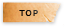# 線性模式分析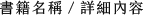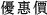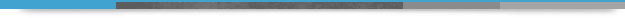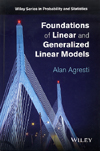## Foundations of Linear and Generalized Linear Models

+作者：

### Agresti

+年份：
2015 年1 版
+ISBN：
9781118730034
+書號：
PS0440H
+規格：

+頁數：
472
+出版商：
John Wiley

\$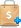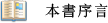A valuable overview of the most important ideas and results in statistical modeling

Written by a highly-experienced author, Foundations of Linear and Generalized Linear Models is a clear and comprehensive guide to the key concepts and results of linearstatistical models. The book presents a broad, in-depth overview of the most commonly usedstatistical models by discussing the theory underlying the models, R software applications,and examples with crafted models to elucidate key ideas and promote practical modelbuilding.

The book begins by illustrating the fundamentals of linear models, such as how the model-fitting projects the data onto a model vector subspace and how orthogonal decompositions of the data yield information about the effects of explanatory variables. Subsequently, the book covers the most popular generalized linear models, which include binomial and multinomial logistic regression for categorical data, and Poisson and negative binomial loglinear models for count data.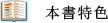●An introduction to quasi-likelihood methods that require weaker distributional assumptions, such as generalized estimating equation methods
●An overview of linear mixed models and generalized linear mixed models with random effects for clustered correlated data, Bayesian modeling, and extensions to handle problematic cases such as high dimensional problems
●Numerous examples that use R software for all text data analyses
●More than 400 exercises for readers to practice and extend the theory, methods, and data analysis
●A supplementary website with datasets for the examples and exercises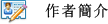Alan Agresti
Distinguished Professor Emeritus
University of Forida
Gainesville, FL1 Introduction to Linear and Generalized Linear Models
2 Linear Models: Least Squares Theory
3 Normal Linear Models: Statistical Inference
4 Generalized Linear Models: Model Fitting and Inference
5 Models for Binary Data
6 Multinomial Response Models
7 Models for Count Data
8 Quasi-Likelihood Methods
9 Modeling Correlated Responses
10 Bayesian Linear and Generalized Linear Modeling
11 Extensions of Generalized Linear Models
Appendix A Supplemental Data Analysis Exercises
Appendix B Solution Outlines for Selected Exercises